### Solar Energy

Everyone is familiar with sunlight: it lets us see during the day, it keeps us warm, and it's an integral part of an enjoyable day at the beach.While we're all familiar with sunlight, the scientific specifics are a bit more complicated. As it turns out, sunlight is electromagnetic radiation from the sun.

This quiz will explore sunlight and electromagnetic radiation in more detail, and provide us with some of the background understanding we need in order to learn how solar energy technologies work and how we can improve their performance.

# EM Basics

Sunlight is electromagnetic radiation, as are other forms of light like the beam from a laser pointer and the glow of a light bulb.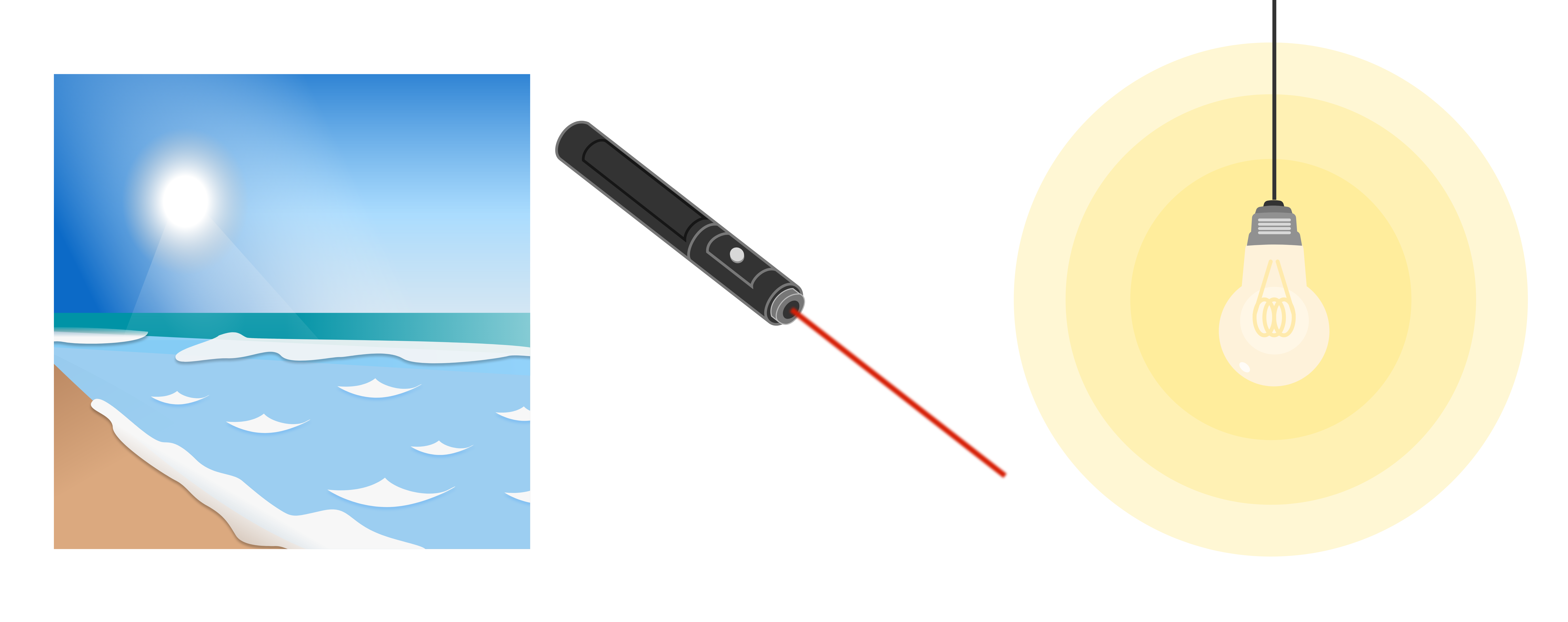Light was a long misunderstood scientific phenomenon, and it puzzled thinkers since the inception of "natural philosophy," when people started studying the world around them in an organized way. Today we have a stronger understanding of light thanks to centuries of scientific inquiry. Starting around the turn of the $19^\text{th}$ century, light was thought of as purely being a wave phenomenon. That is, people started thinking of light as electromagnetic waves. This largely resulted from the work of Thomas Young, an English polymath who developed an early version of the double slit experiment.

# EM Basics

Some scientists before Young (including Newton) believed that light was carried by particles which move in straight lines. This seems reasonable, since a straight line drawn from the edge of a shadow to the source of light will pass through the edge of the object casting the shadow.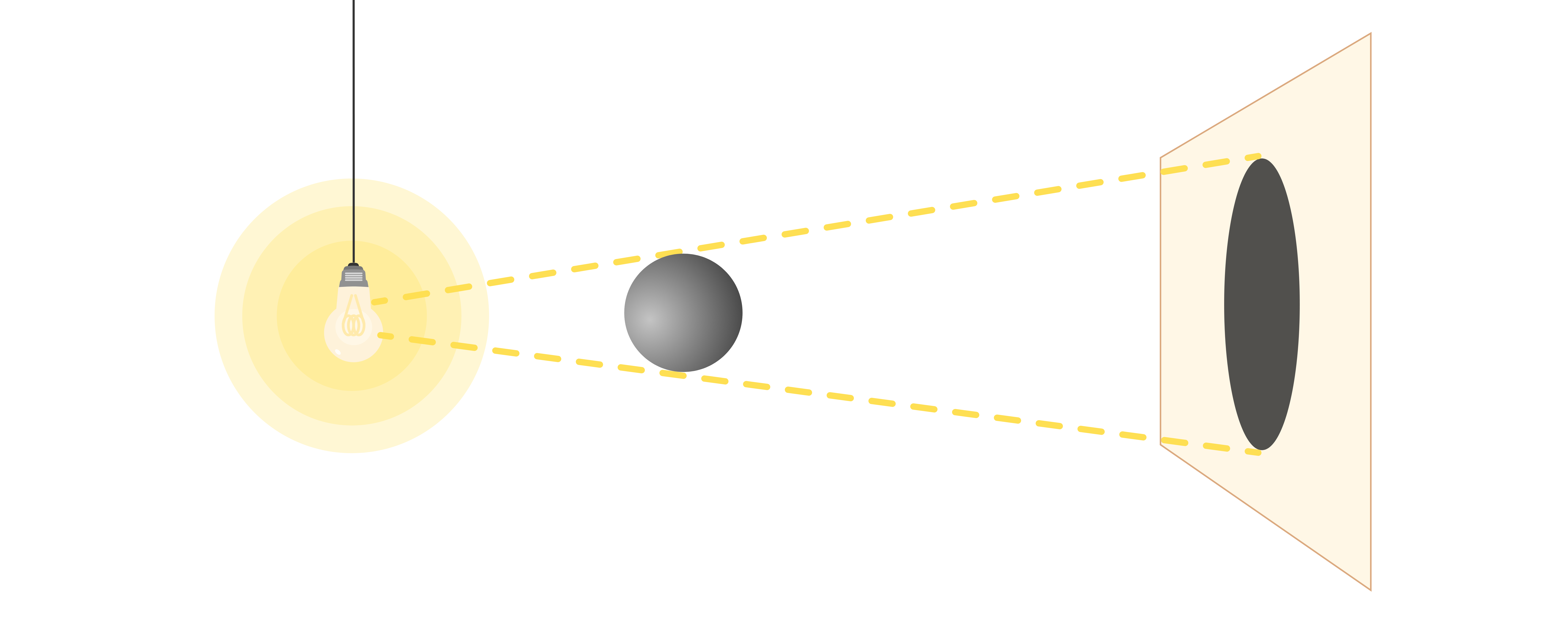Young convinced the other scientists of his day that light traveled as a wave by using a clever setup to show a surprising result. He set up a series of screens as shown in the diagram below. Light was shone through slits in the first two screens to cast a pattern on the third screen.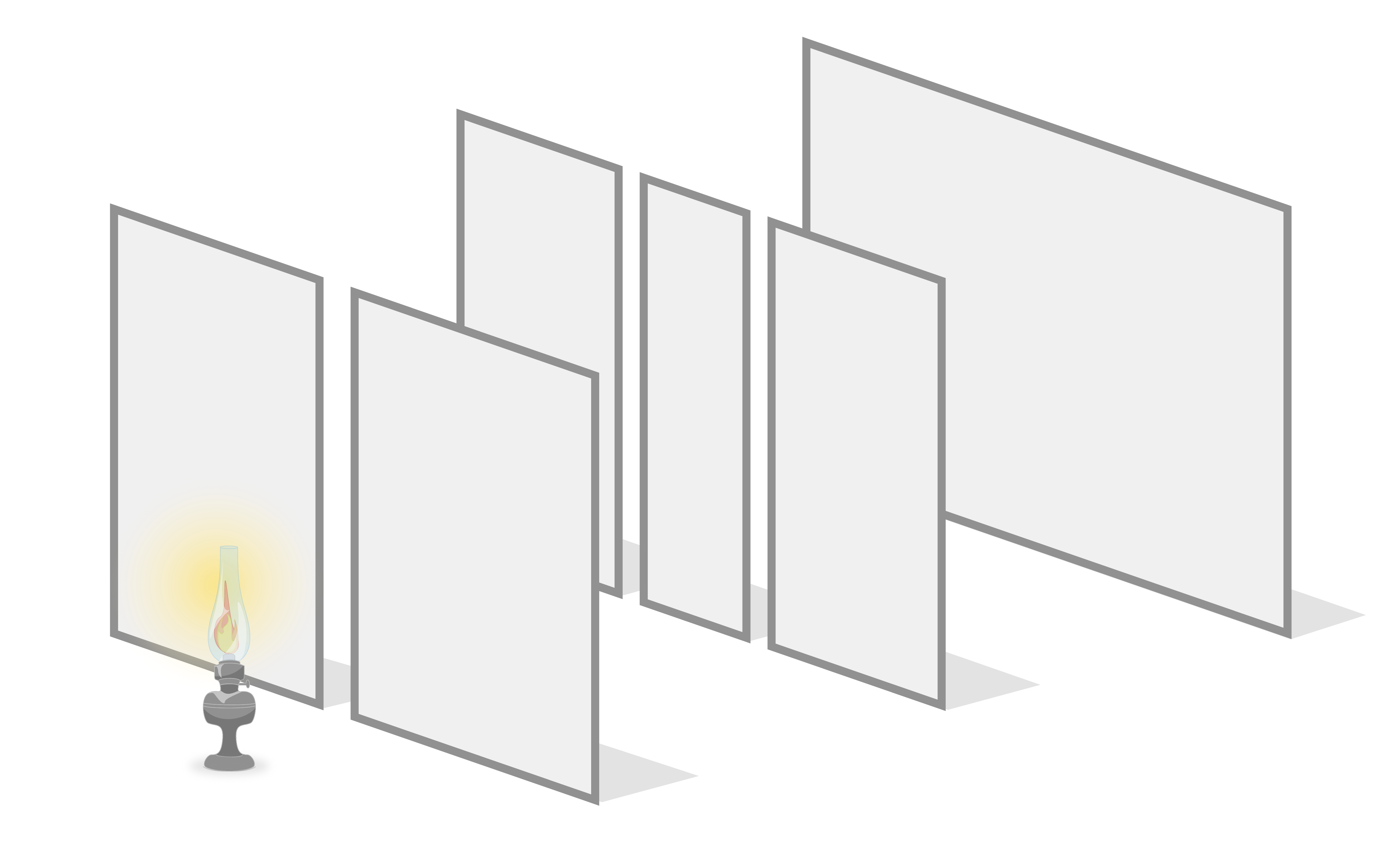If light was carried by particles that moved in straight lines, what light pattern would you expect to see on the last screen?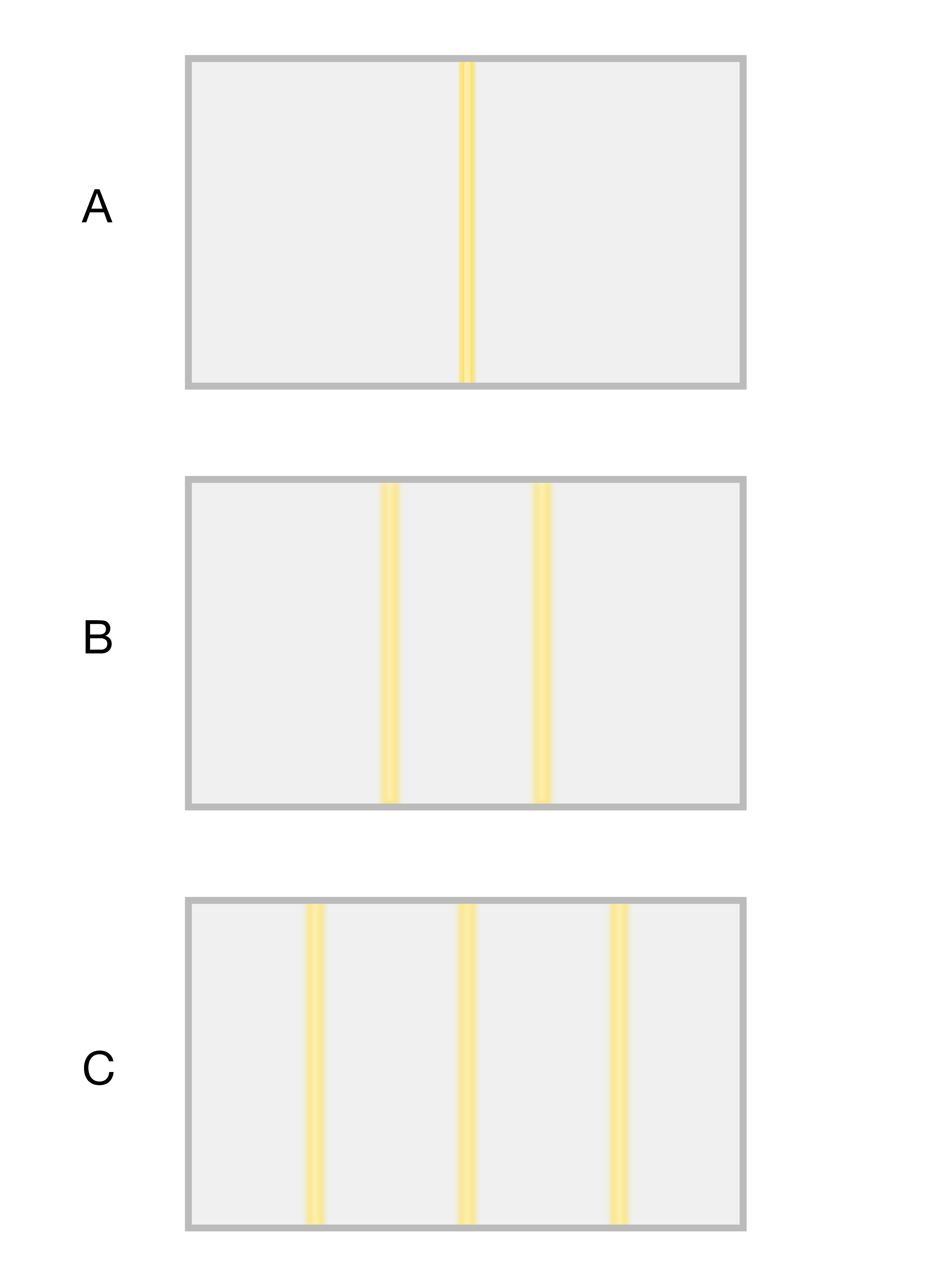# EM Basics

If light was carried by particles moving in straight lines, we’d expect a simple screen pattern with two bright lines. Light would only be able to reach the final screen if it traveled in a straight line from the light source, through the slit in the first screen, and through one of the slits in the second screen. Since there are two slits in the second screen, this gives two possible paths for light to reach the last screen.Surprisingly, this was not what Young observed from his experiment. The result of Young’s experiment was a pattern of alternating bright and dark stripes: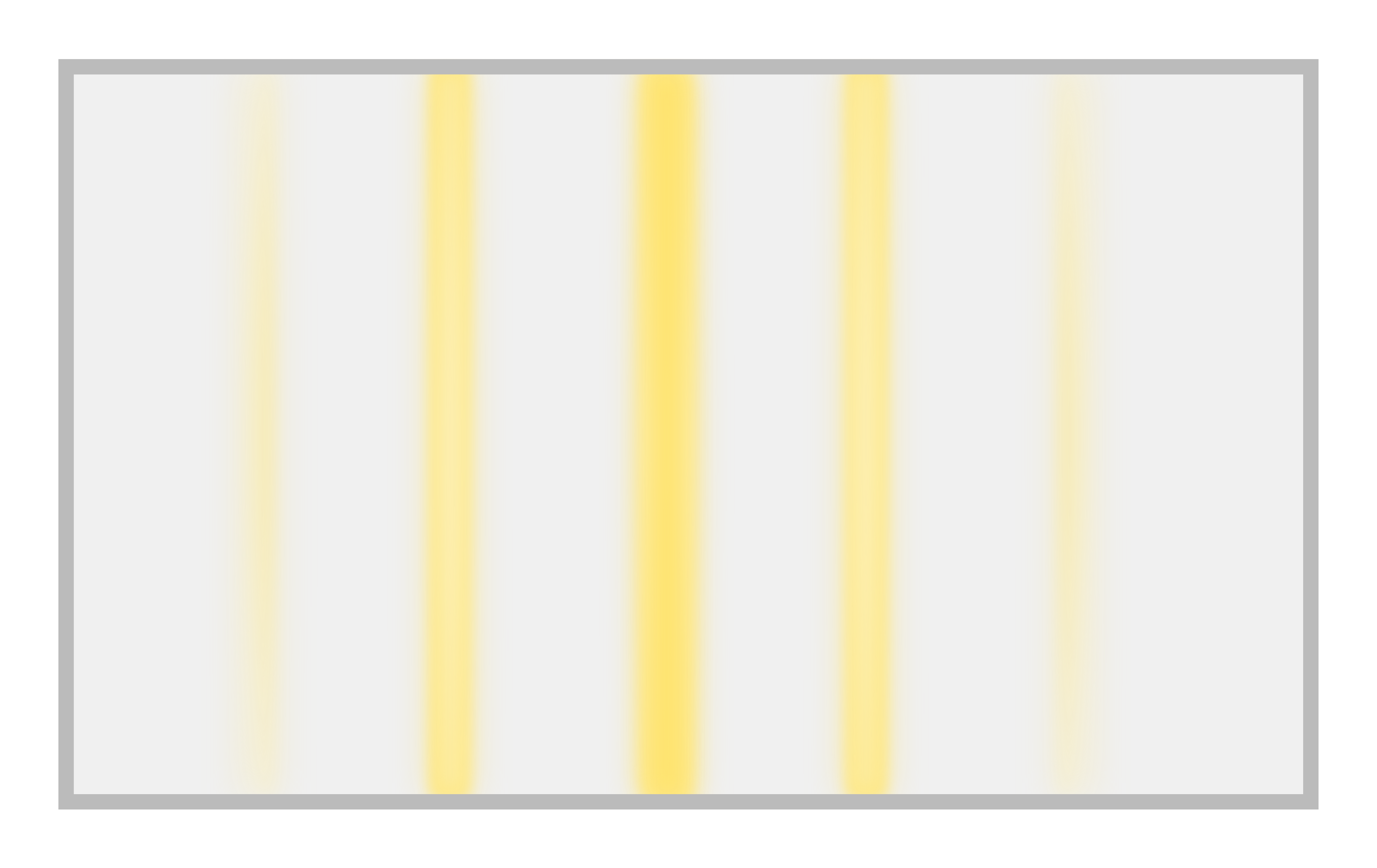This result can be explained if we think of light as being carried by waves rather than particles. Light shining through the first screen creates a point source of waves. When these waves reach the second screen, they can only pass through at the two slits, creating two point sources of waves. These two wave sources interact to create the pattern on the final screen: when the peaks (or valleys) of the waves align, it leads to constructive interference and a bright line. When a peak from one wave aligns with the valley of the other, it leads to destructive interference and a dark line. This is illustrated in the diagram below, which shows Young's experiment from a bird's eye view.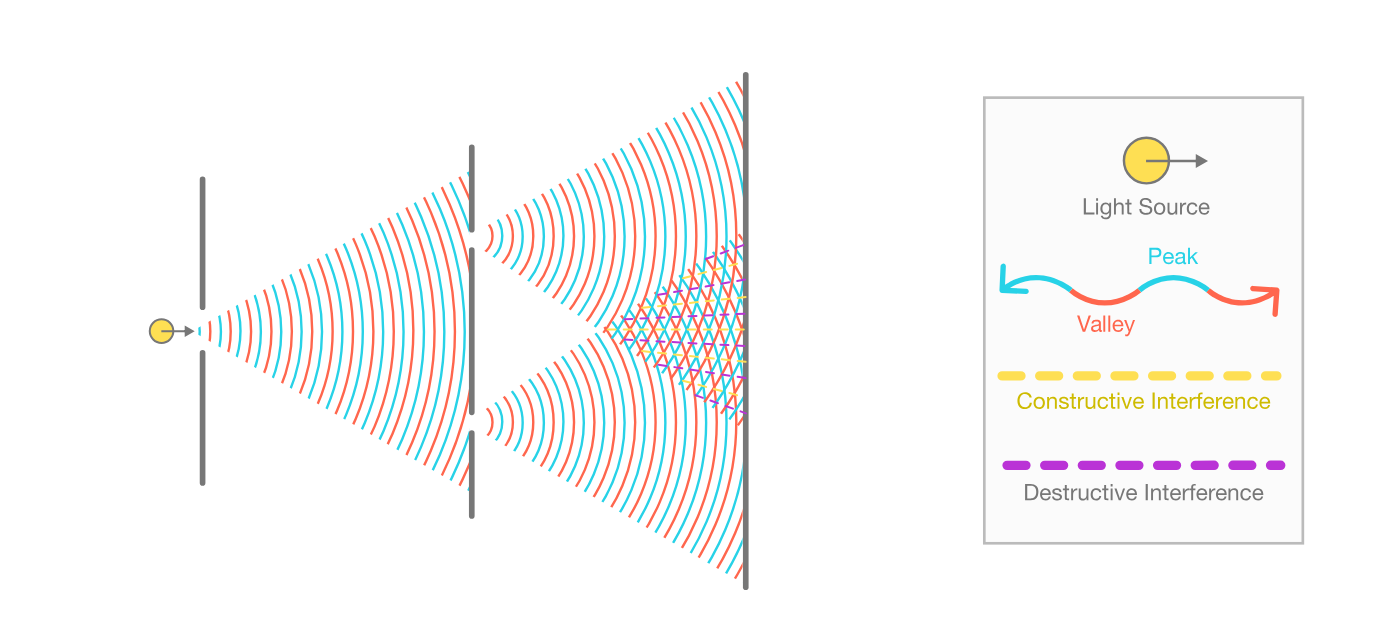With this experiment, Young convinced his contemporaries that light is carried by waves, and because of light's wave-like properties, this experiment remains important to this day. Light being carried by waves gives us some important information about its properties.

# EM Basics

While different types of light are similar in that they are all electromagnetic (EM) radiation, we know from everyday experience that we can tell different types of light apart. This is because EM radiation has properties that can vary between different types of light.EM radiation has some important properties that we know from everyday experience such as intensity and direction. Intensity is a measure of how much energy is being carried by the EM radiation. Direct sunlight is much more intense than candlelight: it is brighter and makes us feel warmer than candlelight does, because it is carrying more energy. EM radiation also has an associated direction that it is traveling in. Even if the results can occasionally be confusing (like in Young's experiment), light travels in a specific direction.

Since we can think of EM radiation as a wave, it also has some relevant wave properties: wavelength and frequency. Light with different wavelengths and frequencies can interact with matter in very different ways.

Note: since EM radiation can be thought of as a wave, you might think that amplitude should also be an important property of light. Light can be thought of as having an amplitude, which would be related to its intensity. Since intensity is more useful and more easily measurable, we typically use intensity rather than amplitude.

# EM Basics

One big difference between sunlight and the light from a lightbulb is their intensity. Colloquially, intensity refers to how bright or how strong a source of electromagnetic radiation is. Scientifically, intensity refers to the density of radiant power carried by the electromagnetic radiation, typically measured in $\si[per-mode=symbol]{\watt\per\meter\squared}$. Intensity is also sometimes called irradiance, and in fact irradiance is the preferred term in SI radiometry units (where intensity means something slightly different). In this course we will use intensity and irradiance interchangeably.

The intensity of sunlight on a sunny day is about $\SI[per-mode=symbol]{1000}{\watt\per\meter\squared}.$ What would the intensity be $($in $\si[per-mode=symbol]{\watt\per\meter\squared})$ on your skin from a $\SI{40}{\watt}$ light bulb that's $\SI{3}{\meter}$ away?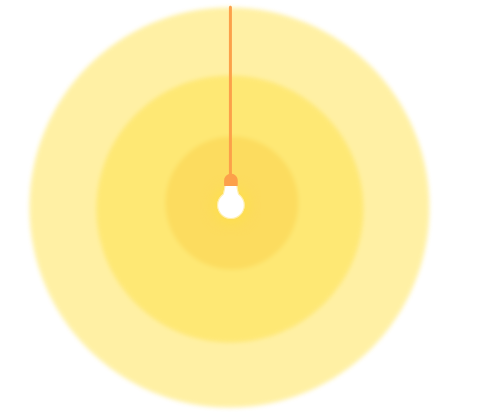Assume that the bulb emits equally in all directions, and that all $\SI{40}{\watt}$ of input power are converted to electromagnetic radiation.

Hint: if you could put a sphere of light collecting material around the lightbulb, you'd collect $\SI{40}{\watt}$ of power, no matter how big the sphere. The surface area of a sphere of radius $r$ is $A = 4\pi r^2.$

# EM Basics

Direction is another property of radiation. As the name suggests, it refers to the direction in which electromagnetic radiation is traveling. Radiation will continue to travel in the same direction until it interacts with matter, which might reflect or scatter it, changing its course.

Sunlight reaching Earth's surface has a relatively uniform direction: the direction is aligned with the vector pointing from the Sun to Earth. Why do you cast a shadow on a sunny day, but not on a cloudy day?# EM Basics

Like all waves, electromagnetic radiation has an associated wavelength. Wavelength is an important property of radiation, and radiation with different wavelengths can interact with matter in very different ways.

Radiation with wavelengths from $\SI{390}{\nano\meter} - \SI{700}{\nano\meter}$ is considered visible light because the human eye responds to those wavelengths. Each wavelength corresponds to a specific color, with the shortest wavelengths being violet light and the longest wavelengths being red light.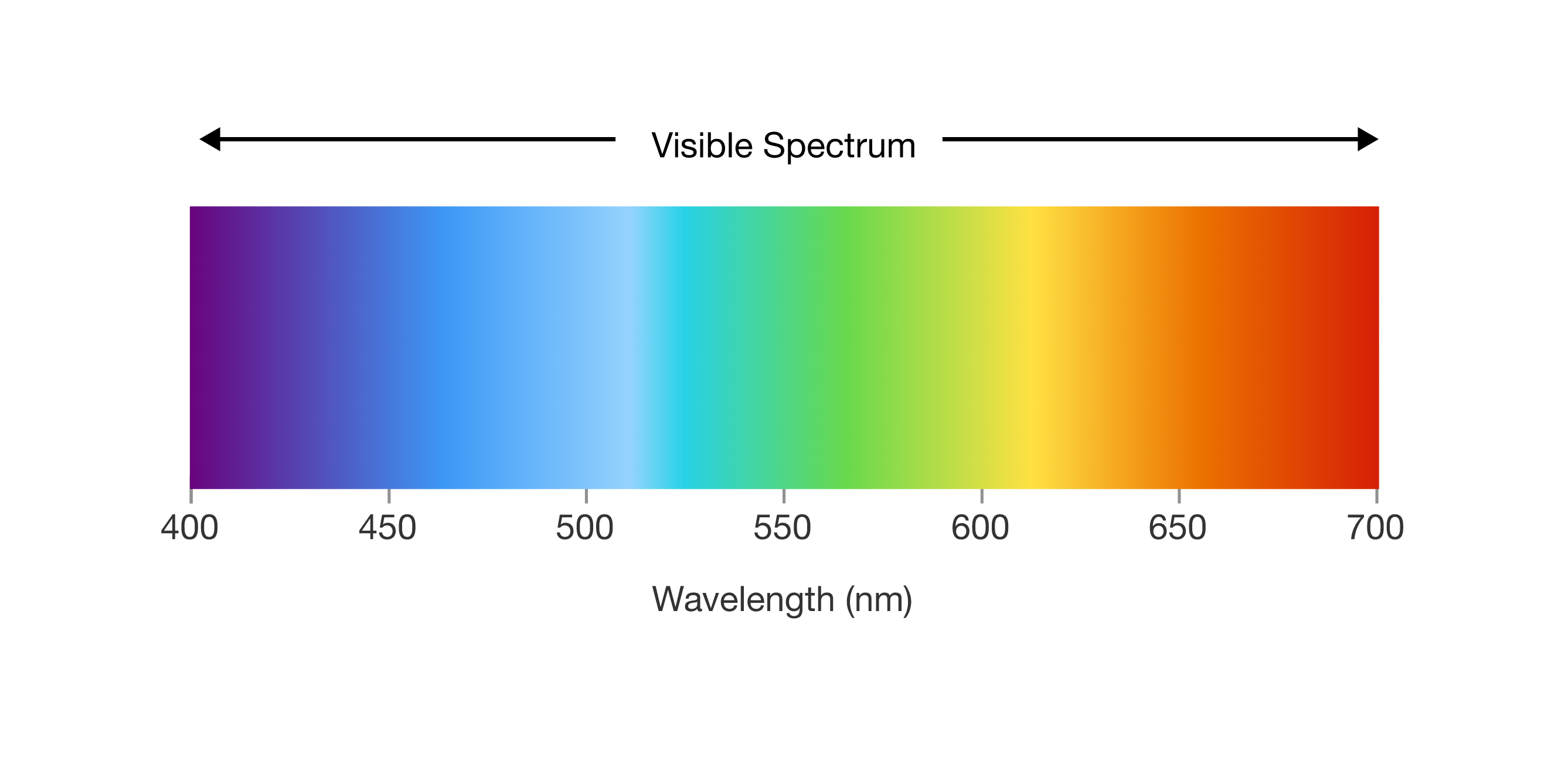Different light sources are sometimes characterized by a "color temperature," measured in Kelvin. Light from cooler objects tends to be more red, while light from hotter objects looks more blue. For example, a campfire might have a color temperature around $\SI{1000}{\kelvin},$ while the sun has a color temperature of about $\SI{6000}{\kelvin}$.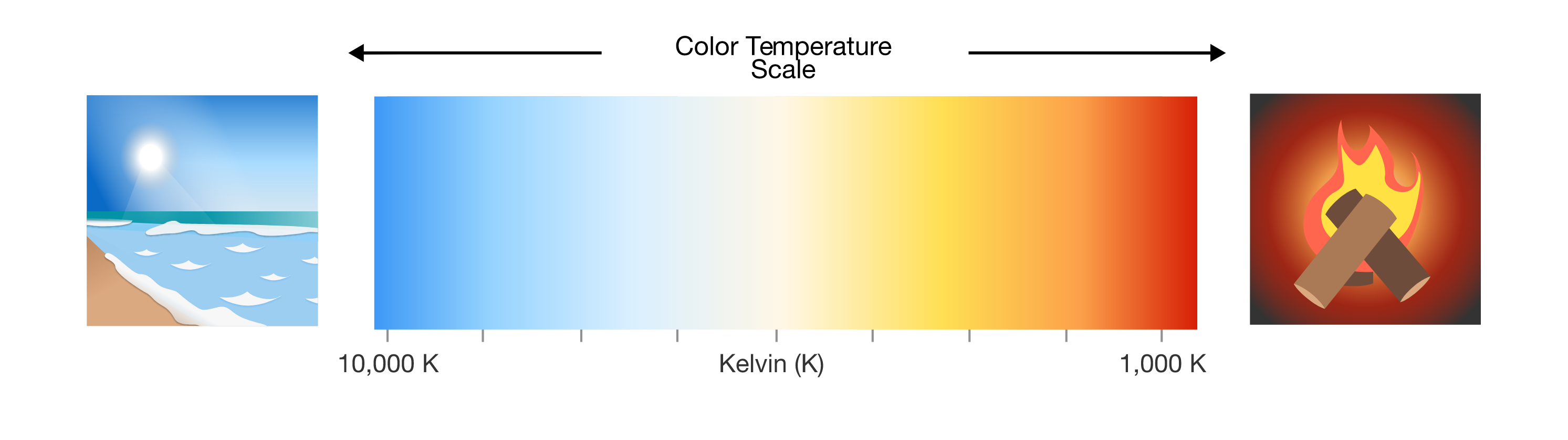Why does a campfire being at a lower temperature lead to it producing a different color of light from the sun?

# EM Basics

The other property of light that comes from its wave like nature is frequency. We know that light behaves like a wave (from Young's experiment), and since light travels through space, light is a type of traveling wave. This is relevant to our discussion on frequency because traveling waves move with a velocity that depends on the wave's wavelength and frequency. A wave travels its wavelength each period, and the number of periods a wave goes through each second is given by its frequency. Therefore, the velocity of a traveling wave is simply the product of its wavelength $(\lambda)$ and frequency $(f):$ $v = \lambda f$ One example of a traveling wave which is very easy to visualize is ripples moving across the surface of a pond. If you throw a stone into a pond, you'll notice that ripples emanate from the point where the stone hits the water. Suppose you throw a stone into a pond and notice that the distance between the ripples is approximately $\SI{10}{\centi\meter}$ and that $4$ ripples hit the edge of the pond each second.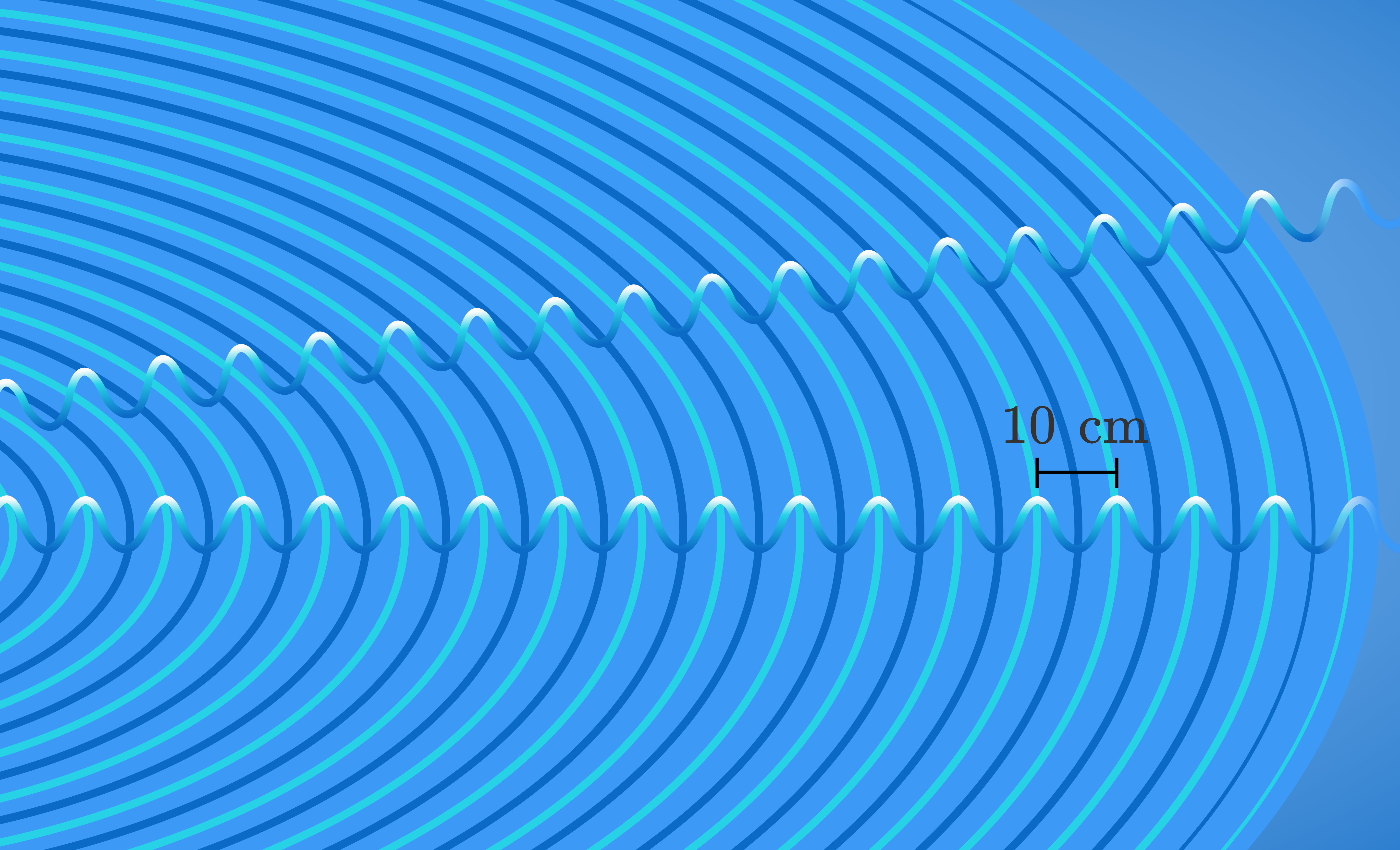What is the approximate velocity of the ripples?

# EM Basics

You might think that light being a traveling wave means it has three properties we should care about: wavelength, frequency, and velocity. While we do care about each of these properties, one of them doesn't vary based on light source: velocity.

Light moving through vacuum (i.e., the absence of matter) travels with the same velocity, regardless of whether that light comes from the sun or from a candle, and regardless of whether it has a wavelength of $\SI{100}{\nano\meter}$ or $\SI{50}{\micro\meter}$. This velocity happens to be the fastest observed velocity in the universe, and is known as the speed of light. The speed of light in vacuum is approximately $\SI[per-mode=symbol]{3e8}{\meter\per\second}$.

For light with a given wavelength, can its frequency vary? Assume the light is traveling in vacuum.

Note: The speed of light does vary depending on the medium it travels through. For example, light in water travels at approximately $\SI[per-mode=symbol]{2.25e8}{\meter\per\second}$.

# EM Basics

Since the speed of light is constant in vacuum, and the frequency and wavelength of a wave can be related through velocity, light of a specific frequency corresponds to a specific wavelength and vice versa. In some situations it might be more convenient to work with the wavelength of light, while in others it might be more convenient to work with the frequency of light. It is uncommon to work with both simultaneously, since giving both the wavelength and frequency of light is superfluous: if you have one you can figure out the other.

Suppose you performed an experiment and observed EM radiation with a wavelength of $\lambda = \SI{500}{\nano\meter}$ $($which we can equivalently write as $\SI{5e-7}{\meter}),$ but you needed to know the frequency of that light rather than its wavelength. What is the frequency of this light?

Remember that the velocity of a wave is related to its wavelength and frequency by: $v = \lambda f$ and that all electromagnetic radiation travels through vacuum at the speed of light $(\SI[per-mode=symbol]{3e8}{\meter\per\second}).$

Note: it is most common for us to observe light in air, not vacuum. Air is a different medium than vacuum, so in principle the speed of light in air can vary from the vacuum value. However, the speed of light in air at standard temperature and pressure differs from the speed of light in vacuum by only $\sim 0.03\%$, so in practice we can essentially always use $\SI[per-mode=symbol]{3e8}{\meter\per\second}$ for the speed of light in air.

# EM Basics

Understanding the properties of intensity, direction, wavelength and frequency lays the foundation for learning how sunlight can be converted into other forms of energy. The wave nature of light gives insight into why light has wavelength and frequency, but it does not accurately capture all the behaviors of light. Even after Young's experiment, unanswered questions still remained and continued to puzzle scientists. The next quiz will introduce the idea of the photon, which answered the remaining questions about light, and is critically important to understanding how PV cells work.

# EM Basics

×